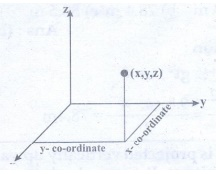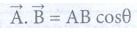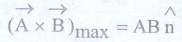Home | | Physics 11th std | Short Questions and Answer

## Chapter: 11th Physics : UNIT 2 : Kinematics

Physics : Kinematics : Book Back Important Questions, Answers, Solutions : Short Questions and Answer

Kinematics | Physics

1. Explain what is meant by Cartesian coordinate system?At any given instant of time, the frame of reference with respect to which the position of the object described interms of position co-ordinates (x, y, z) is called Cartesian Co-ordinate system.

2. Define a vector. Give examples

The physical quantity which is described by both magnitude and direction are called vector. Examples : Force, Velocity, Displacement, Acceleration.

3. Define a scalar. Give examples

The physical quantity which is described only by magnitude are called scalar.

Examples : Distance, Mass, Time, Speed.

4. Write a short note on the scalar product between two vectors.

The scalar product (or) dot product of two vectors is defined as the product of the magnitude of both the vectors and the cosine of the angle between them.5. Write a short note on vector product between two vectors.

The vector product (or) cross product of two vectors is defined as another vectors having a magnitude equal to the product of the magnitude of two vectors and the sine of the angle between them.6. How do you deduce that two vectors are perpendicular?

(i) The scalar product of two vectors.then these two vectors are perpendicular to each other because cos 90° = 0, then.= AB cos θ = AB cos90° = 0.

Then the vectorsandare said to be mutually orthogonal.

(ii) The vector product of two vectors will have maximum magnitude when sin 90° = 1

i.e. θ = 90° thenthen the vectorsandare orthogonal to each other.

7. Define displacement and distance.

Distance : Distance is the actual path length travelled by an object in the given interval of time.

Displacement : Displacement is the difference between the final and initial positions of the object in a given interval of time.

8. Define velocity and speed.

Velocity: Velocity is equal to rate of change of position vector with respect to time.

Velocity is a vector quantity,Speed: The speed is defined as the ratio of total path length travelled by the particle in a time interval. It is a scalar quantity.

Speed = Path length / Time

9. Define acceleration.

● The acceleration of the particle at an instant is equal to rate of change of Velocity.

● Acceleration is a vector quantity. Its SI unit is ms-2 and its dimentional formula is10. What is the difference between velocity and average velocity.Velocity

1. Velocity is equal to rate of change of position vector with respect to time.

2. It tells about the direction of motion of the particle.

Average Velocity

1. The average velocity is defined as rate of the displacement vector to the corresponding time interval.

2. The direction of average velocity is in the direction of displacement vector

One radian is the angle substended at the center of a circle by an arc that is equal in length to the radius of the circle.

12. Define angular displacement and angular velocity.

i) Angular displacement: The angle described by particle about the axis, of rotation in a given time is called angular displacement. The unit of angular displacement is radian. Angular displacement θ = s / r

ii) Angular Velocity : The rate of change of angular displacement is called angular velocity.

The unit of angular velocity is radian per second Angular Velocity ω = dθ / dt

13. What is non uniform circular motion?

The velocity changes in both speed and direction during the circular motion is called non - uniform circular motion.

14. Write down the kinematic equations for angular motion.

i) ω = ω0 + α t

ii) θ = ω0 t + ½ α t2

iii) ω2 = ω02 + 2αθ

iv) θ = [ (ω0)t ] / 2

15. Write down the expression for angle made by resultant acceleration and radius vector in the non uniform circular motion.

Centripetal acceleration = v2 / r

Magnitude of resultant acceleration

aR = √ [ at2 + (v2/r)2 ]The resultant acceleration makes an angle θ with radius vector

The angle is given by tan θ = at / (v2 / r)

Tags : Kinematics | Physics , 11th Physics : UNIT 2 : Kinematics
Study Material, Lecturing Notes, Assignment, Reference, Wiki description explanation, brief detail
11th Physics : UNIT 2 : Kinematics : Short Questions and Answer | Kinematics | Physics